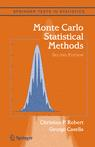Want to share your content on R-bloggers? click here if you have a blog, or here if you don't.Edward Kao pointed out several typos in Example 5.18 of Monte Carlo Statistical Methods. First, the customers in area i should be double-indexed, i.e.$Z_{ij}simmathcal{M}(1,(p_1,ldots,p_5))$

which implies in turn that$T_i=sum_{j=1}^{n_j}Z_{ij}$.

Then the summary T should be defined as$mathbf{T}=(T_1,T_2,ldots,T_n)$

and$W_5$ as$W_5=sum_{i=m+1}^nT_{i5},$

given that the first m customers have the fifth plan missing.